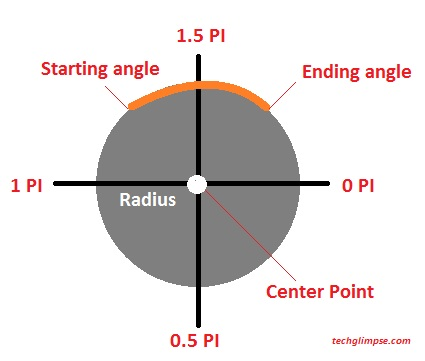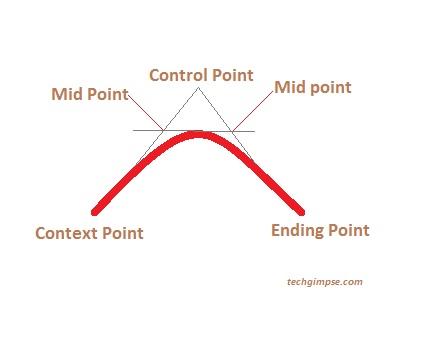1. Home
2. Programming Tools
3. Draw Arc, Quadractic and Bezier Curve in HTML5 Canvas – [Basics]

# Draw Arc, Quadractic and Bezier Curve in HTML5 Canvas – [Basics]

Last week I wrote a tutorial on how to draw a Line, Circle and Custom shapes in HTML5 Canvas. We also learnt how to apply gradient fills to shapes. Today, lets see about Arc, Quadratic and Bezier Curves.

## Arc

In order to create an arc, you can use arc() method and the parameters are center point, radius, a starting angle, an ending angle and drawing direction (which is anticlockwise or clockwise). Once the arc is created, you can set width using lineWidth property and set color using strokeStyle.

Have a look at the picture which explains the parameters.Now consider an imaginary circle which can be defined by x,y coordinates and a radius. Arc is something that lies over an imaginary circle that starts at one point (startAngle) and ends at one point (endAngle). The angles are defined in radians. Finally, the direction at which the arc should be drawn; antiClockwise defaults to false when not specified.

Lets see the code now!

```<canvas id="arc" width="400" height="250"></canvas>
<script>
var canvas = document.getElementById('arc');
var context = canvas.getContext('2d');
var x = canvas.width / 2;
var y = canvas.height / 2;
var startAngle = 1.1 * Math.PI;
var endAngle = 1.9 * Math.PI;
context.beginPath();
context.arc(x, y, radius, startAngle, endAngle, false);
context.lineWidth = 20;
context.strokeStyle = '#e12a2b';
context.stroke();
</script>```

##Quadratic Curve can be created using quadraticCurveTo() method which consists of a starting point or context point, ending point and control point. The control point defines the curvature. For instance, moving the control point away from context and ending point will provide sharp curve.To create a quadratic curve, you have to moveTo() a position to start the context point and use quadraticCurveTo() with the above said parameters.

Have a look at the code.

```<canvas id="qCurve" width="400" height="200"></canvas>
<script>
var canvas = document.getElementById('qCurve');
var context = canvas.getContext('2d');
//Create the path first
context.beginPath();
//Move to a position to create context point
context.moveTo(200, 150);
context.lineWidth = 20;
context.strokeStyle = '#e12a2b';
context.stroke();
</script>```

## Bezier Curve

Bezier Curve is formed by context point, two control points and ending point. To create the curve, you can use bezierCurveTo() method. The code will look almost similar to quadratic curve, with two extra parameters.

```<canvas id="bCurve" width="400" height="200"></canvas>
<script>
var canvas = document.getElementById('bCurve');
var context = canvas.getContext('2d');
context.beginPath();
context.moveTo(200, 130);
context.bezierCurveTo(180, 40, 400, 40, 360, 140);
context.lineWidth = 10;
context.strokeStyle = '#e12a2b';
context.stroke();
</script>```Learn to draw Custom Shapes and Gradient fills in Canvas

Updated on October 16, 2017